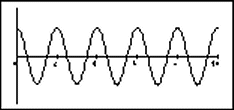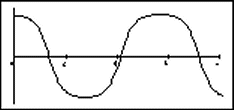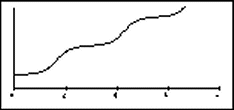Teaching Physics with the Physics Suite Edward F. Redish

Pendulum graphs

Some of the graphs shown below represent the motion of a pendulum -- a massive ball attached to a rigid, nearly massless rod, which in turn is attached to a rigid, nearly frictionless pivot.

On the right below are shown four graphs of the pendulum's angle as a function of time. On the left are a set of four initial conditions and a denial. Match each graph with its most likely initial conditions (or with the denial). Note that the scales on the y axes are not necessarily the same. (There is not necessarily a one-to-one match.)

 (1) θ0 = 120o ω0 = 0  (2) θ0 = 173o ω0 = 30o/s  (3) θ0 = 6o ω0 = 0  (4) θ0 = 173o ω0 = 0  (5) Not a possible pendulum graph. (A)(B)(C)(D)Not finding what you wanted? Check the Site Map for more information.